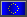Mouser Europe

Incoterms: DDP is available to customers in EU Member States.
All prices include duty and customs fees on select shipping methods.

Incoterms: DDU applies to most non-EU customers.
Duty, customs fees and taxes are collected at time of delivery.

 Euros Euros are accepted for payment only in EU member states these countries.

 US Dollars USD is accepted in all countries. Other currency options may also be available - see Mouser Worldwide.

•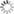•••••••••••••••••# Bench Talk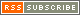### Bench Talk for Design Engineers | The Official Blog of Mouser Electronics

Solve the Mystery of Vehicle Detection Algorithm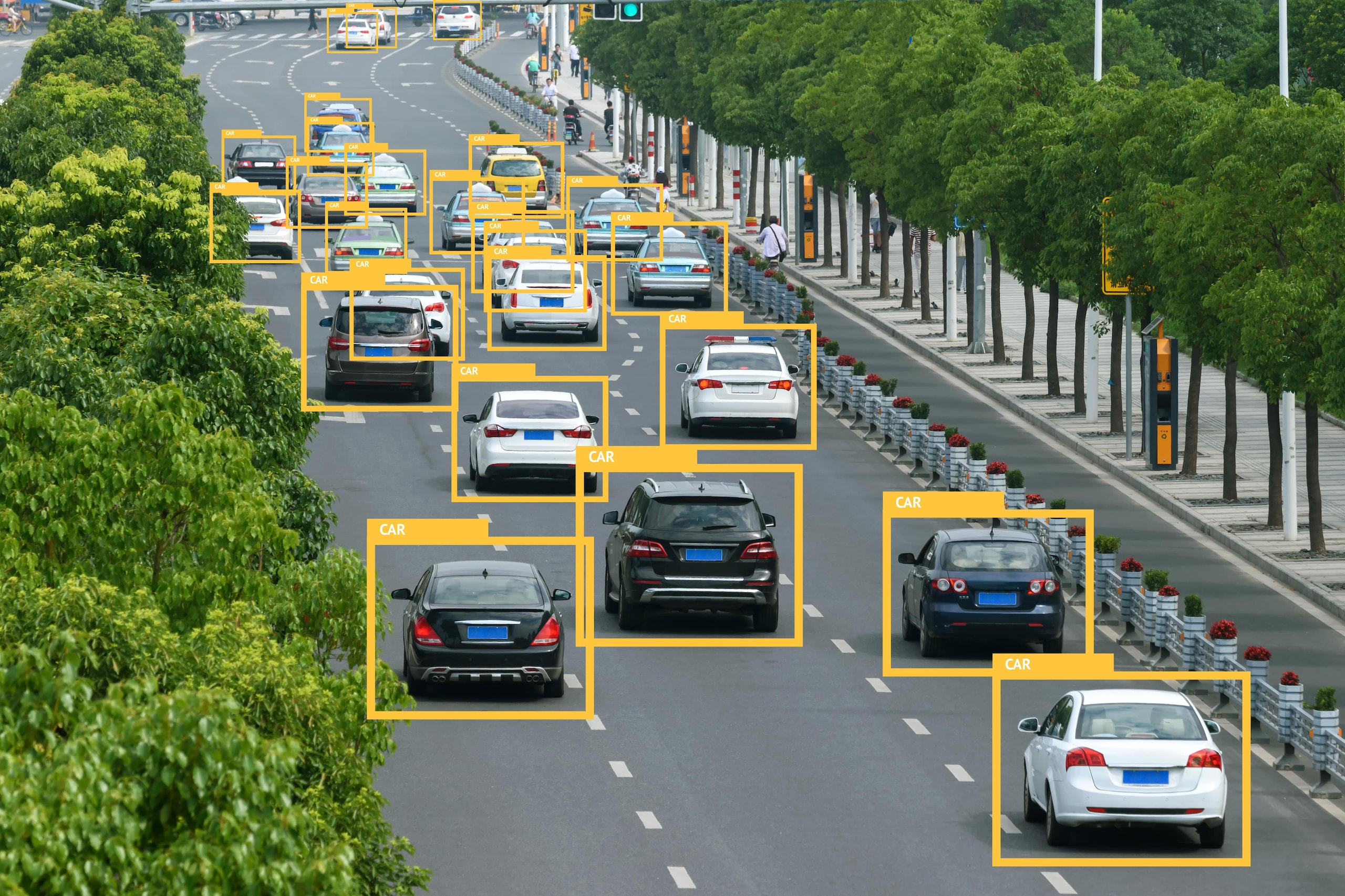(Source: Zapp2Photo/Shutterstock.com)

As mysterious as vehicle detection might seem, the technology essentially boils down to a mathematical formula that calculates the pixel features of a specified area of an image and then determines the category to which the object belongs based on the corresponding features. Object detection methods can generally be broken down into the two steps of feature extraction and category determination, with the commonly used methods of support vector machines (SVMs) and histograms of oriented gradients (HOGs) being used in coordination with each other.

This article will introduce commonly used vehicle-detection algorithms, focusing on the aspects listed below to unravel the mysteries behind these algorithms and to provide the reader with a clear understanding of the machine learning process.

• Overview of Application Scenarios
• A Detailed Description of HOG Feature Calculations
• An Overview of the SVM Workflow
• Comparison and Summary

## Overview of Application Scenarios

Vehicle-detection technology is widely used in the real world as illustrated in the following examples. The personal cars we drive on a regular basis sometimes have one or more onboard rearview cameras. The system is activated when another vehicle is about to pass within a certain distance to the rear. Once a vehicle is detected to the rear of the vehicle, an alert is issued and the driver is prompted to slow down (Figure 1). Another example can be found in applications pertaining to the field of automated driving, in which positions of surrounding cars are used to analyze their speeds, distances, and other factors before automatically adjusting the car's path in response.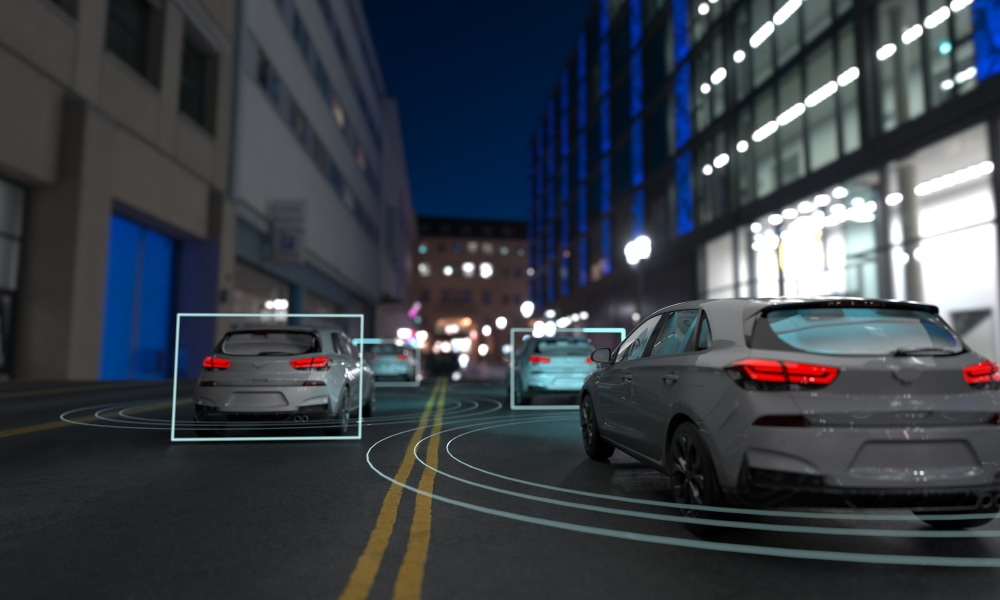Figure 1: A rearview camera can detect vehicles within the image and indicates the vehicles' positions using rectangular boxes. (Source: Just Super/Shutterstock.com)

Vehicle-detection systems are also widely used for traffic control and road condition monitoring (Figure 2). For example, the system pictured is placed at a tunnel entrance and counts daily traffic flow during a given time period and implements the corresponding restriction policies appropriately, thus reducing the occurrence of traffic accidents and providing information to drivers regarding where traffic is congested or light. This allows drivers to choose the best route for avoiding traffic. In addition, traffic-flow statistics can also be used by parking lots found in airports or train stations, with big-data analysis used to determine whether parking spaces are in high demand so that staff can respond and allocate resources accordingly.

Signals produced by vehicle detection systems can be combined with other technologies to detect traffic flow around a given traffic signal, and workers can leverage big data and artificial intelligence to calculate and determine a reasonable time interval for traffic lights.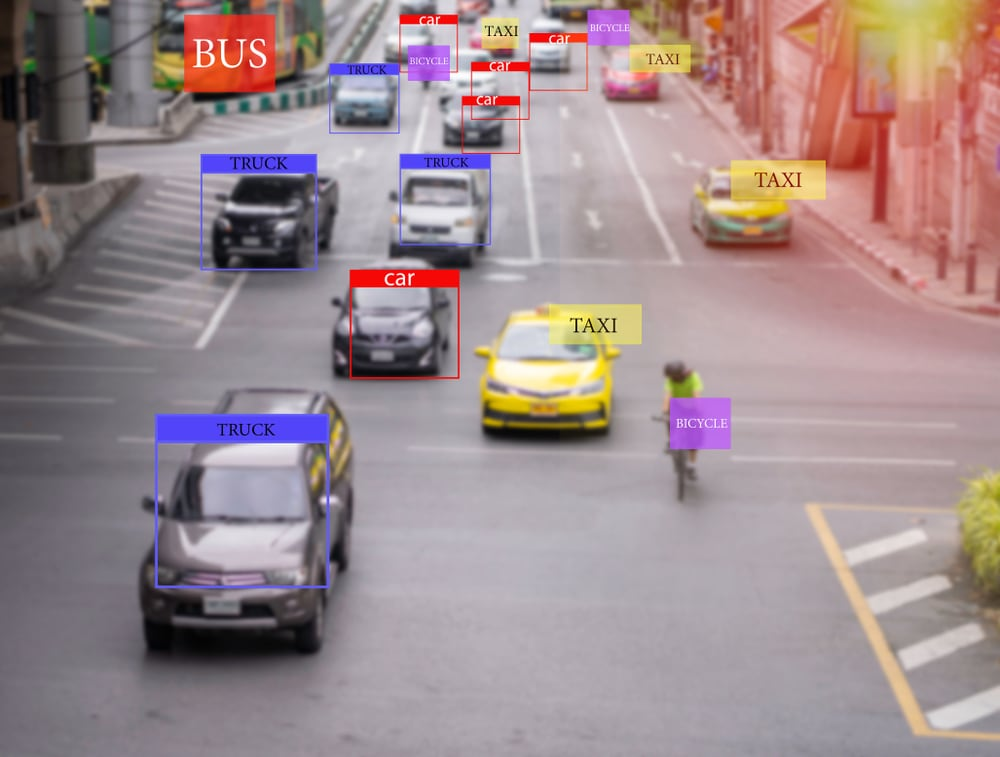Figure 2: Application of a vehicle detection system in a road condition monitoring scenario. (Source: PaO_STUDIO/Shutterstock.com)

## Joint HOG and SVM Algorithms

(40 − 16) / 8 + 1 = 4

A method for the detection of pedestrians using HOG combined with SVM was originally proposed by the French researcher Dalal in 2005 at the Conference on Computer Vision and Pattern Recognition in San Diego, Calif. Now the HOG+SVM approach has evolved to detect all kinds of objects, including the locations of vehicles and traffic lanes.

## HOG Feature Calculation

1. HOG is a local feature extraction algorithm, and so this algorithm will not serve the purpose of detecting an object in a large image containing a complex background even if more features are extracted. The image needs to be cropped to be able to acquire the target object. It has been experimentally proven that the vehicle used as the target object must account for more than 80 percent of the image in order to obtain favorable results. The cropped local image is partitioned into blocks, and each block is extracted for features composed of individual cells.

(In each image, multiple pixels form a single cell, and multiple cells make up a block.)

The HOG feature calculation process is explained using Figure 3 below as an example.

First, the entire picture is cropped to obtain a 40px x 40px image, after which we must define the following variables: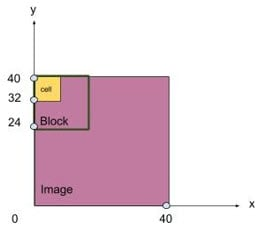Figure 3: To illustrate the movement of a block in a cropped image, this figure shows that 4 cells form a block with a side length of 16px, and the image is then cropped to obtain a length and width of 40px x 40px. The corresponding step size is 1, meaning that movement is carried out one pixel at a time. (Source: Original artwork created for Mouser)

1. We define the movement step s, such as: s =1.
2. We further define the cell size in pixels, such as: 8 x 8.
3. We further define the block size, such as: each block consists of 2 x 2 = 4 cells.
4. Finally, we define the number of bins, setting the value as needed, such as: bin = 9. Each bin is used to store the calculated histogram gradient direction's accumulated value, as further explained below.

2. The input image and color are standardized to reduce the interference of light or shadow on the detection accuracy of objects in the image, which is done by performing a gamma color correction and changing the image to grayscale (the principles underlying gamma color correction are ignored for the purposes of this article).

3. The size of the gradient is calculated.

The calculation method is explained using an example of a partial block belonging to a cell (Figure 4), and the formula used to calculate the midpoint for a pixel value of 25 is shown as follows.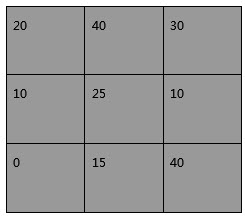Figure 4: Partial block size and pixel values for a single cell. (Source: Original artwork created for Mouser)

Using a reasonable definition based on a convolutional kernel approach, we can show experimentally that [-1, 0, 1] works best. The convolution kernel [-1, 0, 1] can be understood as a matrix used to calculate the gradient amplitude direction for each pixel. Therefore, we can use [-1, 0, 1] for the horizontal (x-axis positive direction, rightward) and [-1, 0, 1]T for the vertical (y-axis positive direction, upward) to perform horizontal and vertical gradient component calculations for each pixel in the image area. The squared sum and the root sign of the two gives the direction of the gradient at that point, and thus the formula used is as follows: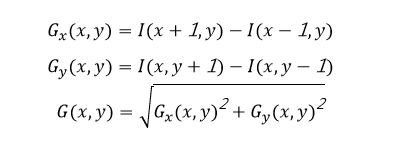Therefore, the horizontal direction of a point with pixel value 25 is calculated as shown in Figure 5 below: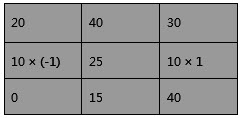Figure 5: Calculating the pixel value for the horizontal direction of a midpoint with pixel value 25. (Source: Original artwork created for Mouser)

Formula: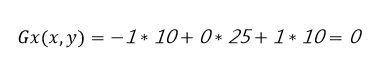Therefore, the vertical orientation of a point with a pixel value of 25 is calculated as shown in Figure 6: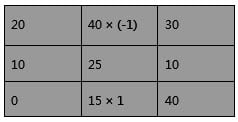Figure 6: Calculating the pixel value for the horizontal direction of a midpoint with pixel value 25. (Source: Original artwork created for Mouser)

Formula: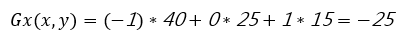4. The corresponding gradient direction is calculated using the following formula: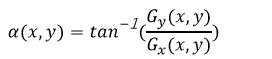5. By repeating Steps 3 through 4 in the calculation process for all the pixels in each cell and summing the values, we obtain a gradient integration plot in nine gradient directions for each cell (Figure 7).

6. We solve for the HOG features of the image block, meaning that we concatenate the included cell features together.

7. We then solve for the HOG feature of the whole image, meaning that we concatenate the included image block features.

8. Method of calculating feature dimensions:

The block in the above example is moved four steps each in both the x and y directions:

(40-16)/8+1=4

Each block includes four cells:

2*2=4

Formula for calculating feature dimensions: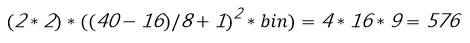Thus, the feature dimension calculated for the current image example corresponds to 576.

9. We then normalize the obtained gradient vector. The key goal of normalization is to prevent overfitting, which can result in good classification of the training set but extremely low test set detection rates, a situation that is obviously unacceptable for our purposes. Using the same approach we use for the normalization of machine-learning features, if we obtain an eigenvalue distributed between (0, 200), for example, we need to prevent numbers under 200 from affecting the overall distribution of features (the model will deviate from the overall trend to fit 200, resulting in overfitting), so we need to normalize the feature distribution to a certain interval. Dalal mentions in his paper that the results obtained using L2-norm are highly satisfactory.

(Here, 0, 200 represents the range of the eigenvalue)

10. Features along with corresponding labels are sent to the SVM to train the classifier.

## Histogram Gradient Direction and Bin Values

Dalal mentions in his paper that "the purpose of this step is to provide an indication of the direction of the quantization gradient of the function for the local image region while remaining able to maintain weak sensitivity to the appearance of the detected object in the image."

The gradient size is inserted into the corresponding bin based on the direction of the gradient, and there are two possible methods for defining direction.

An unsigned approach is suitable for vehicle or other object detection, while a signed approach has been experimentally proven to be unsuitable for vehicle or other object detection. However, this approach can be useful when the image is zoomed in, zoomed out, or rotated, after which the pixels are returned to their original positions. Refer to the fifth link included in the references for a more in-depth look.

1. Unsigned: (0, π)

Below we take a closer look at unsigned interpolation.

In this article, we will use three tables to explain how interpolation is carried out. In each table, the first row shows the calculated amplitude and the second row specifies the directional value of the bin which is obtained by dividing 180 degrees by the number of defined bins. The third row shows the bin sequence numbers, starting with 0.

The image can be split into as many bins as necessary. For example, when it is divided into nine bins, i.e., when a gradient histogram with nine directions per cell is used, each bin covers an area of 20 degrees. Amplitudes (calculated above) are inserted into each bin, and the final sum of the amplitudes in each bin corresponds to the vertical axis of the histogram, while the horizontal axis corresponds to the range of bin values, in this case (0, 8).

## Interpolation Method:

If a pixel has an amplitude of 80 and an orientation of 20 degrees, these values are inserted into the corresponding positions in the blue area of Table 1.

Table 1: Values to insert in the corresponding positions if a pixel has an amplitude of 80 and an orientation of 20 degrees.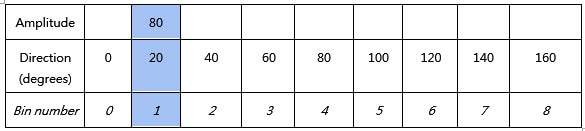If the amplitude is 80 and the direction is 10 degrees, then these values are inserted separately into the two positions in the blue areas in Table 2.

Table 2: Values to insert in the corresponding positions if a pixel has an amplitude of 80 and an orientation of 10 degrees.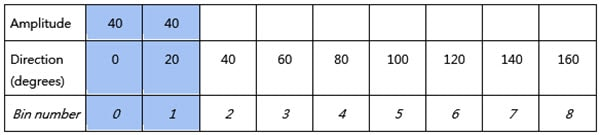If the amplitude is 60 and the direction is 165 degrees, then these values are separately inserted into the two positions in the blue areas in Table 3.

(180 degrees and 0 degrees are equivalent in direction, so the amplitude is inserted into two bins at a ratio of 1:3 each)

Table 3: Values to insert in the corresponding positions if the amplitude is 60 and the direction is 165 degrees.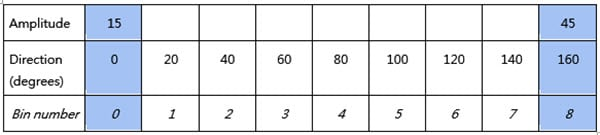Table 1 above shows the interpolation method used when the direction value is exactly the same as the corresponding value of the bin, Table 2 shows the interpolation method used when the direction value falls between two bin values, and Table 3 shows the interpolation method used when the direction value is greater than the maximum bin value. According to the principles underlying the three methods when performing calculations using cells as the unit of measure, the amplitude of all pixels in a cell is accumulated after traversal. For example, in the sample above we obtained two amplitude values of 40 and 15 at bin 0, so our histogram for bin 0 has so far accumulated up to 55. The amplitudes of each cell from bins 1 through 8 are calculated and summed in the same manner. We ultimately end up with a histogram similar to the following (Figure 7), with horizontal coordinate X indicating gradient direction and vertical coordinate Y indicating gradient amplitude.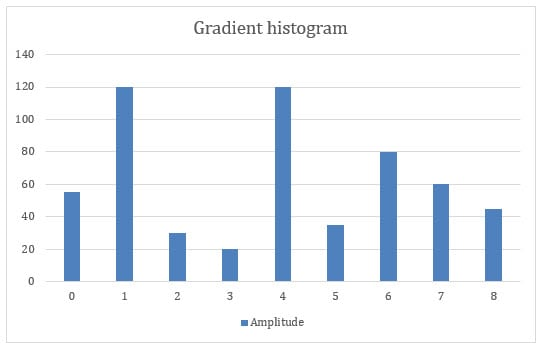Figure 7: An example of a gradient histogram: The horizontal axis corresponds to the bin number, and the vertical axis corresponds to the calculated amplitude. The numbers corresponding to the vertical axis of the figure are given for illustrative purposes only. For example, the bin 1 amplitude values in the three tables above are summed to get 80 + 40 + 0 = 120 as the final result, and we obtain 120 for the histogram vertical coordinates. As we continue to calculate the value of bin 1, the value will continue to accumulate. (Source: Original artwork created for Mouser)

Experimental results have shown that the best results are obtained when using nine bins for target detection and unidirectional interpolation.

2. Signed: (0, 2π)

When adding a plus or minus sign before the directional value, if nine bins are also defined, then the angular range assigned to each bin is: (0, π/9°).  For example, for the second bin, which has a positive value interpolated into the range 20–40 (the blue sector), a negative value should be interpolated into the 200–220 bin (the blue sector). (Figure 8)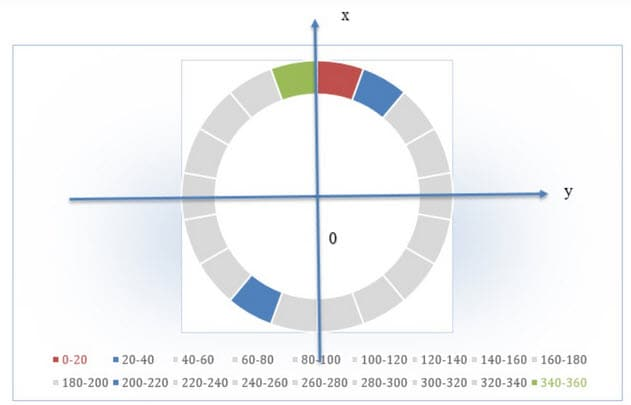Figure 8: In signed interpolation, each sector represents a bin coverage angle range value. Red corresponds to bin number one, which covers 0 to 20 degrees. Moving clockwise in order, green marks the end at 340–360 degrees. The blue area indicates the corresponding bins in both directions. (Source: Original artwork created for Mouser)

## An Overview of the SVM Workflow

An SVM (support vector machine) separates two classes in space through a hyperplane, and the two-dimensional space can be understood simply as the space where y is found and y satisfies: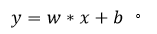The y value determines whether the sample is classified as positive or negative. However, in order to determine the optimal hyperplane, here we introduce support vectors and maximum intervals. Our goal is to find a hyperplane such that there is maximum separation between the points closest to the hyperplane (Figure 9).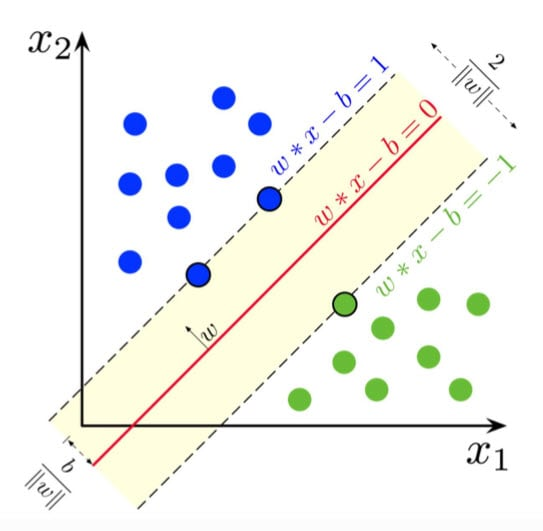Figure 9: The red line corresponds to the hyperplane, the points on either side of the dashed line are support vectors, and the calculated value is 1 (positive class) or -1 (negative class). The blue point corresponds to a positive sample, and the green point corresponds to a negative sample. The goal is to find the value exhibiting the largest distance between the dashed lines, since a larger distance indicates a better binary classification model. (Source: Wikipedia)

Because of the high complexity of data presented in real situations, kernel functions are sometimes introduced as needed in order to map low dimensionality onto high dimensionality and render linearly inseparable data (Figure 10) linearly separable by finding the optimal hyperplane.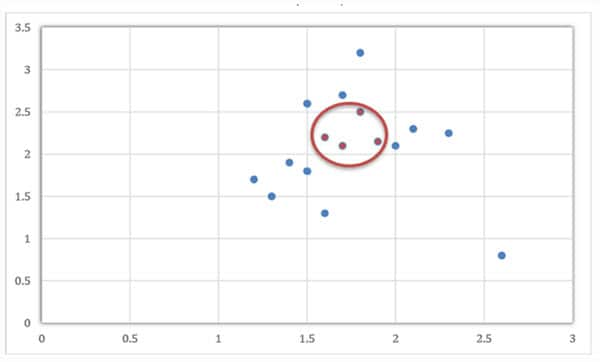Figure 10: The points in the red circle are linearly inseparable from the points in blue in two-dimensional space, so a kernel function is needed to map the points into a higher-dimensional coordinate system. (Source: Original artwork created for Mouser)

SVMs are computationally intensive and time-consuming to train because the similarity to every other point is computed for each point. Therefore, SVMs are suitable for training binary classification models with small amounts of data, and if multiple categories are involved, multiple models are usually trained separately. In addition, two open-source tools developed by professors at National Taiwan University are now very popular among scientists. The first is LibSVM, and the other one is Liblinear, which was developed based on SVM techniques for large amounts of data.

SVMs are extremely parameter-sensitive. During the training of LibSVM or LibLinear, it is important to pay close attention to the penalty term C and the weighting factor w. C is the penalty term and the larger it is, the better the classification effect during the training process. However, when C is too large, overfitting can occur, meaning that training sample classification accuracy will be extremely high but test accuracy will be extremely low. There will inevitably exist data points that lie far away from the central cluster of the set, and the size of C indicates our willingness to drop these outliers. A larger value for C indicates we are reluctant to discard these outliers, so the model would fit particularly well to the training set but not the test set. W, or weight, represents the coefficient for positive and negative samples, and if we want more targets to be detected, we can increase the positive sample weight. However, doing so will result in a false detection rate (FP) that is particularly high. Conversely, an increased negative sample weight will reduce the false detection rate (FP), but the target detection rate (TP) will naturally also decrease.

A simple experiment carried out by the author found that using a million data points and 1152-dimensional features, it took 20 minutes to open 18 threads for training using two CPUs and 60G of RAM while running Windows 10. Therefore, it is recommended to either use the Liblinear library to train on a very large corpus or increase the available computer memory.

## Comparison and Summary

In this article, we focused on understanding feature calculation in the context of vehicle detection and briefly explored the SVM classification strategy. When HOG features are used for vehicle detection, the use of unsigned interpolation of features with more than 1,000 dimensions in nine bins is recommended, and an uneven feature distribution must be normalized. For SVM, kernel functions can be selected as needed, and the LibSVM library can be used to train models based on very large amounts of data if necessary.  The SVM's kernel function mechanism for mapping low dimensional spaces to high dimensional spaces effectively solves the problem of linear inseparability. The computational complexity of the SVM is determined by the number of support vectors and the final decision function is fortunately determined by a small number of support vectors. SVMs also have their limitations. If you omit the use of the LiBSVM/Liblinear open-source library, the processing of large amounts of data can be very difficult when using SVM alone. This is because the SVM calculation process involves matrix calculations, and the number of rows and columns is determined by the number of samples. Therefore, large samples consume a lot of time and space during the calculation process. Similarly, in practice, the choice of whether or not to leverage HOG should be based on a two-way consideration of the technique's advantages and disadvantages. These advantages and disadvantages are summarized here for the reader's reference.

• Advantages: HOG is done on a local unit basis, which can better capture local shape information while ignoring lighting, color and other factors. For example, the color of a car can be ignored during vehicle detection, thus reducing the required number of feature dimensions, and because of the technique's weak sensitivity to light, vehicles can still be detected even when partially blocked from view.

• Disadvantages: HOG is not very good at dealing with occlusion, and changes in vehicle direction are not easy to detect. Because of the nature of the gradient, HOG is quite sensitive to noise, so after blocks and cells are split into local area units, it is often necessary in practice to perform Gaussian smoothing to remove noise. The determination of feature dimensions (cell, block, step size) is very demanding, and in practice, it is necessary to make multiple attempts to achieve an optimal solution.

I hope that this article has given you a clearer idea of the current state of the art in vehicle detection. Although combining SVM and HOG is computationally intensive, the corresponding cost is low, and training of the model can be done on a standard CPU, which makes the use of this approach very popular among small and medium-sized product development companies. For example, the development and output of small parts such as onboard cameras is likely to continue to yield better price-performance while also ensuring an acceptable level of usability.

« Back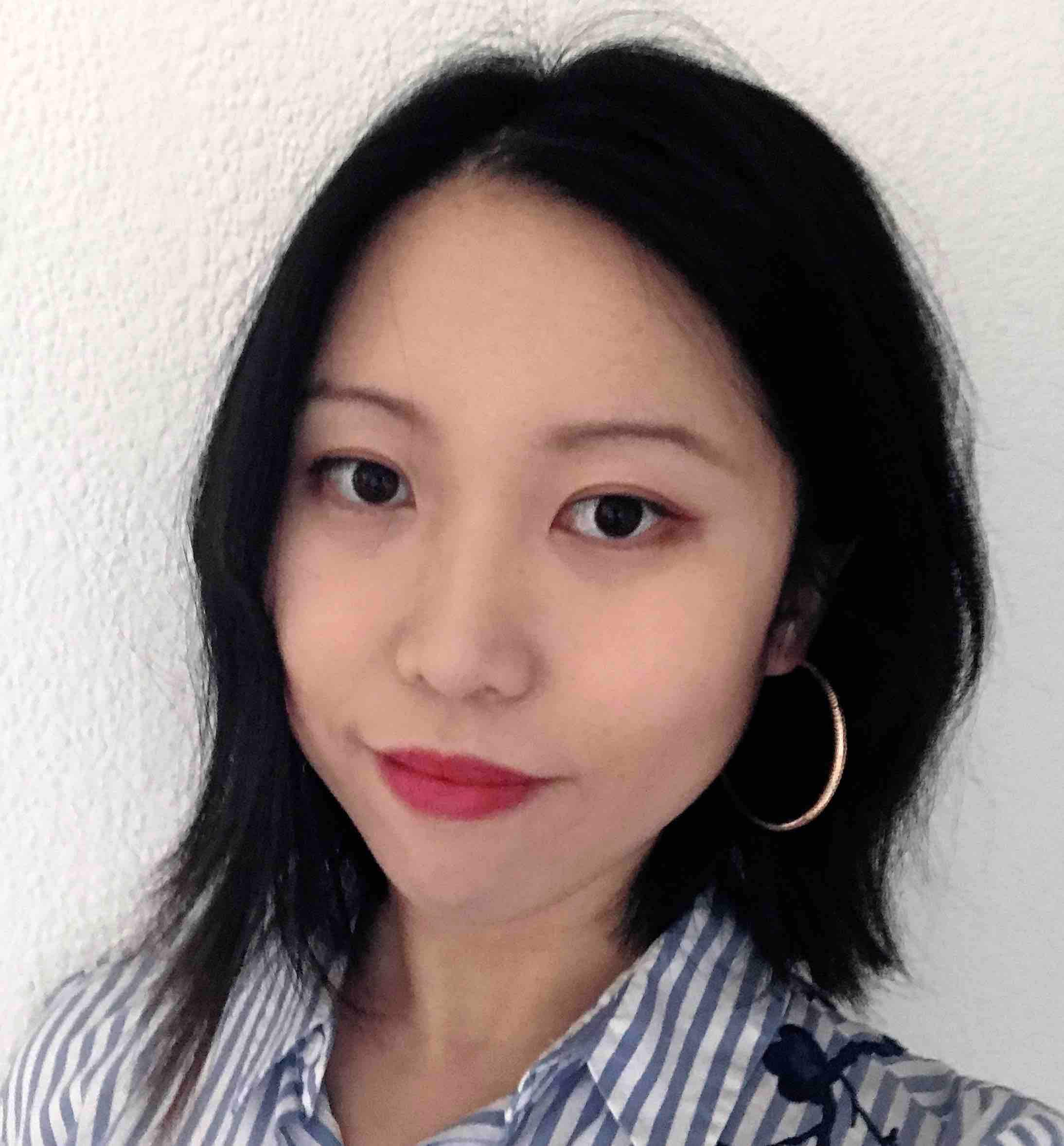Wang Jing is a machine-learning algorithm engineer currently working in the field of automotive inspection. Passionate about creating technical articles, she hopes her writings will arouse readers' interest in artificial intelligence and inspire more professionals to combine AI with cloud technology and big data to make life safe and convenient.